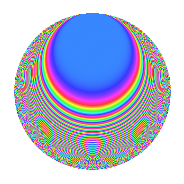# Properties

 Label 51.2.iLevel 51 Weight 2 Character orbit i Rep. character $$\chi_{51}(5,\cdot)$$ Character field $$\Q(\zeta_{16})$$ Dimension 32 Newform subspaces 1 Sturm bound 12 Trace bound 0

# Related objects

## Defining parameters

 Level: $$N$$ = $$51 = 3 \cdot 17$$ Weight: $$k$$ = $$2$$ Character orbit: $$[\chi]$$ = 51.i (of order $$16$$ and degree $$8$$) Character conductor: $$\operatorname{cond}(\chi)$$ = $$51$$ Character field: $$\Q(\zeta_{16})$$ Newform subspaces: $$1$$ Sturm bound: $$12$$ Trace bound: $$0$$

## Dimensions

The following table gives the dimensions of various subspaces of $$M_{2}(51, [\chi])$$.

Total New Old
Modular forms 64 64 0
Cusp forms 32 32 0
Eisenstein series 32 32 0

## Trace form

 $$32q - 8q^{3} - 16q^{4} - 8q^{6} - 16q^{7} - 8q^{9} + O(q^{10})$$ $$32q - 8q^{3} - 16q^{4} - 8q^{6} - 16q^{7} - 8q^{9} - 16q^{10} + 16q^{12} - 16q^{13} + 16q^{15} + 16q^{18} - 16q^{19} + 16q^{21} - 16q^{22} + 16q^{24} + 16q^{25} - 8q^{27} + 32q^{28} - 8q^{30} + 16q^{31} + 96q^{34} + 8q^{36} + 16q^{37} - 24q^{39} + 16q^{40} - 56q^{42} + 16q^{43} - 40q^{45} - 32q^{46} - 64q^{48} - 48q^{49} - 40q^{51} - 96q^{52} - 24q^{54} - 48q^{55} + 8q^{57} - 48q^{58} + 32q^{60} - 32q^{61} + 64q^{63} + 16q^{64} + 72q^{66} + 80q^{69} + 48q^{70} + 64q^{72} + 48q^{73} + 88q^{75} + 48q^{76} + 96q^{78} + 16q^{79} + 48q^{81} + 112q^{82} - 56q^{87} + 16q^{88} - 88q^{90} + 16q^{91} - 72q^{93} - 48q^{94} - 112q^{96} - 16q^{97} - 64q^{99} + O(q^{100})$$

## Decomposition of $$S_{2}^{\mathrm{new}}(51, [\chi])$$ into newform subspaces

Label Dim. $$A$$ Field CM Traces $q$-expansion
$$a_2$$ $$a_3$$ $$a_5$$ $$a_7$$
51.2.i.a $$32$$ $$0.407$$ None $$0$$ $$-8$$ $$0$$ $$-16$$

## Hecke Characteristic Polynomials

There are no characteristic polynomials of Hecke operators in the database